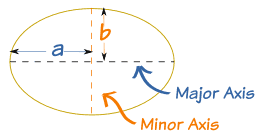## Ellipse Volume Perimeter Area

 Area of Ellipse Enter Radius a = b =
 Result: Area of Ellipse Perimeter of Ellipse

## Ellipsoid Volume Calculator

 Volume of Ellipsoid

Ellipse Calculator to find out the Ellipse Perimeter and Area of the given values of Radius R1 and R2.

The Rectangle Calculator also could calculate the Volume of Ellipsoid for the given values of radius R1, R2 and R3. Ellipse is a regular oval shape in geometry.

Ellipse fig### Ellipse Formulas

Ellipsoid Volume = (4π/3) a b c

Ellipse Perimeter = 2π (√(a2 + b2) / 2)

Ellipse Area = π a b